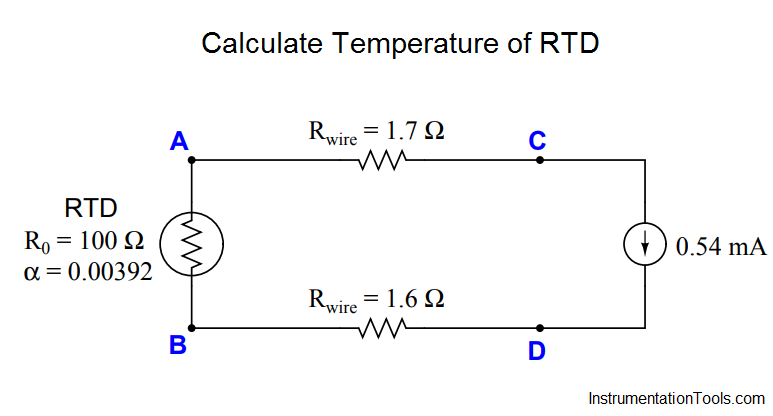# Calculate Temperature of RTD

Determine the temperature of the RTD, given a measured voltage of −59.7 millivolts between test points C and D in this circuit:Assume a 100 Ω RTD with α = 0.00392.

RRTD = 107.256 Ω
T = 65 degrees Fahrenheit (from table)
T = 65.32 degrees Fahrenheit (from formula)